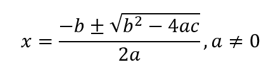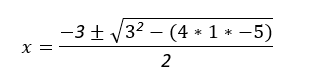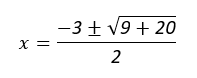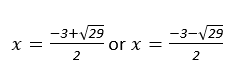PRIMARY

2 - 6

SECONDARY

7 - 10

SENIOR

11 - 12

Home

Theory & Practice Question.

## Theory

When a quadractic equation of the form ax² + bx + c = 0 cannot be
factorised, the quadractic formula can be used to solve for x :Note: If b² - 4ac < 0 there is no solution.

## Example

Solve the following equation for  using the quadratic formula:

+3x-5=0

## Solution

Recall the quadratic formula for the quadratic ax² + bx + c = 0Therefore:

+3x-5=0

a=1 b=3 c=-5

Substituting values: